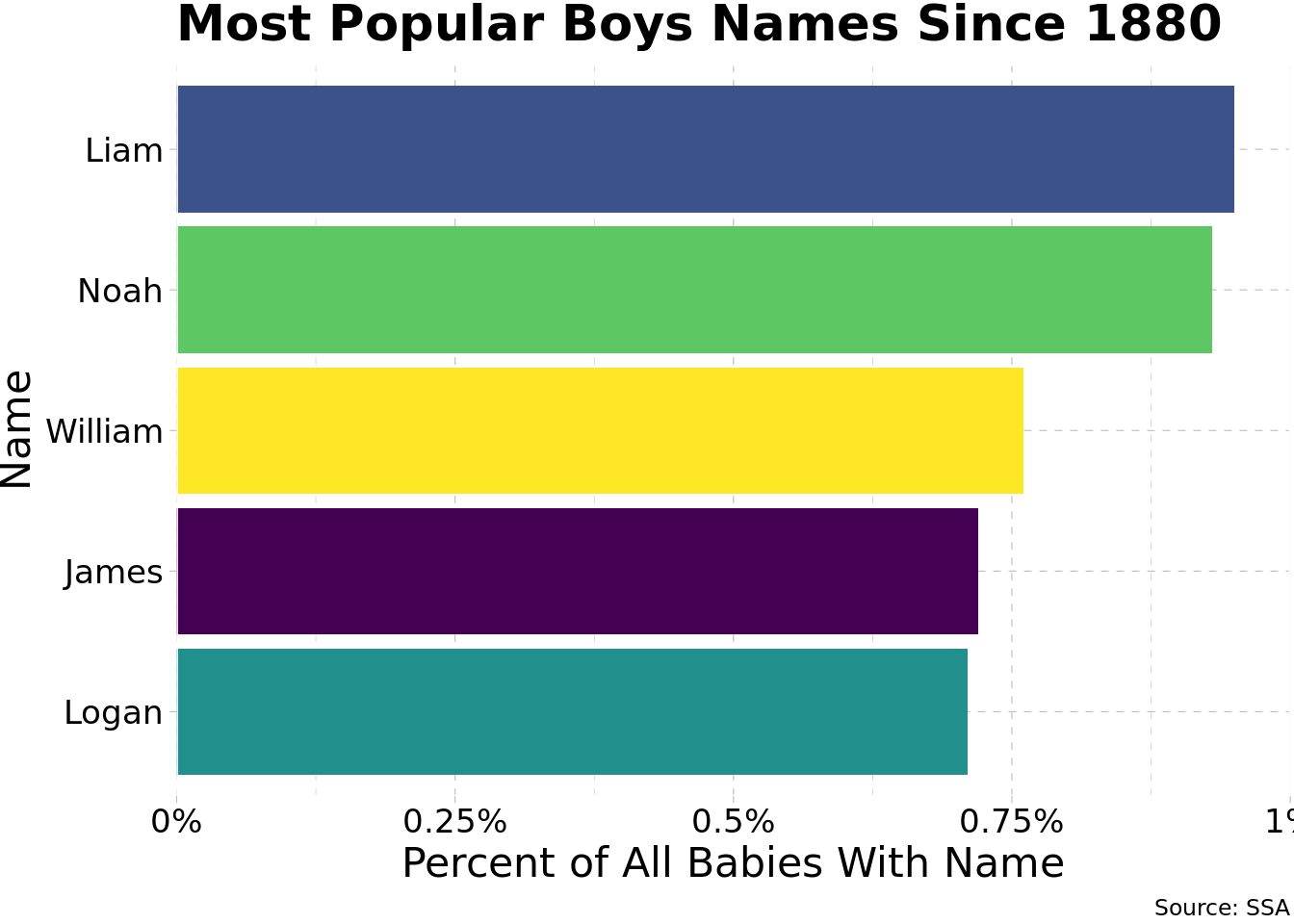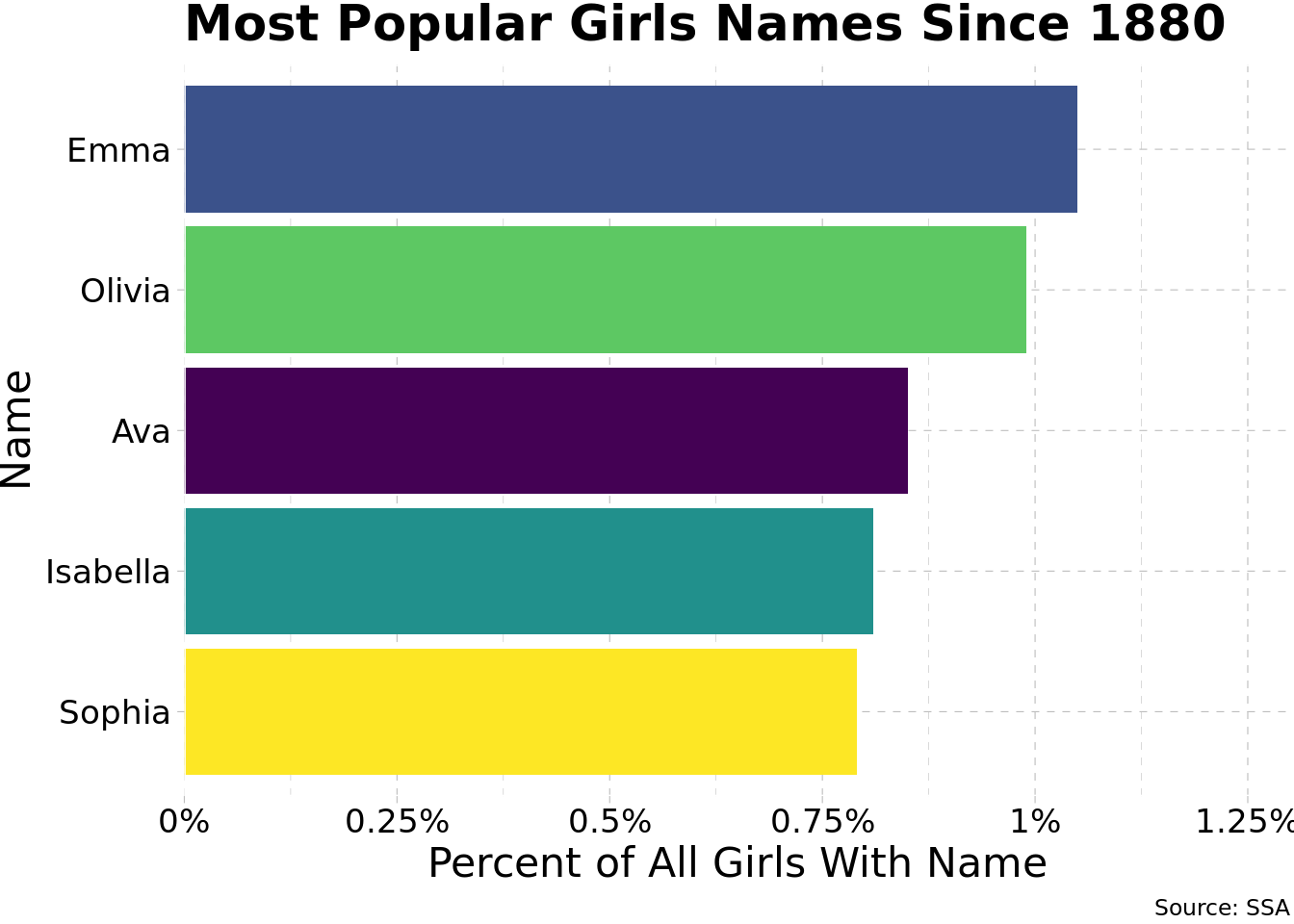# Problem Set 1

Author

Published

September 21, 2022

# The Popularity of Baby Names

Install and load the package `babynames`. Get help for `?babynames` to see what the data includes. Also, don’t forget to load `tidyverse`!

``````# write your code here!
# install.packages("babynames") # install for first use
# Note I've “commented” out some of these commands  (with a #) so they do not run when I run this chunk or render this document

library(tidyverse) # load for data wrangling``````
``````── Attaching packages ─────────────────────────────────────── tidyverse 1.3.2 ──
✔ ggplot2 3.3.6      ✔ purrr   0.3.4
✔ tibble  3.1.8      ✔ dplyr   1.0.10
✔ tidyr   1.2.1      ✔ stringr 1.4.1
✔ readr   2.1.2      ✔ forcats 0.5.2
── Conflicts ────────────────────────────────────────── tidyverse_conflicts() ──

## Question 1

### Part A

What are the top 5 boys names for 2017, and what percent (note not the proportion!) of overall names is each?

``````# write your code here!

# save as a new tibble
top_5_boys_2017 <- babynames %>% # take data
filter(sex == "M", # filter by males
year == 2017) %>% # and for 2007
arrange(desc(n)) %>% # arrange in largest-to-smallest order of n (number)
slice(1:5) %>% # optional, look only at first 5 rows; head(n=5) also works
mutate(percent = round(prop*100, 2)) # also optional, make a percent variable rounded to 2 decimals

# look at our new tibble
top_5_boys_2017``````

The top 5 names are

``````top_5_boys_2017 %>%
select(name,percent) %>%
knitr::kable() # for nicer table in rendered document``````
name percent
Liam 0.95
Noah 0.93
William 0.76
James 0.72
Logan 0.71

Alternatively, you could just write what you found manually into an object like:

``````top_5_boys_2017_alt <- c("Liam", "Noah", "William", "James", "Logan")

top_5_boys_2017_alt``````
`` "Liam"    "Noah"    "William" "James"   "Logan"  ``
``````# you could alternatively add a command,
# %>% pull(name) to the first chunk of code,
# and it would do the same thing, but we'd want to save it,
# for example:

top_5_boys_2017_alt <- babynames %>%
filter(sex=="M",
year==2017) %>%
arrange(desc(n)) %>%
slice(1:5) %>%
mutate(percent = round(prop*100, 2)) %>%
pull(name)

top_5_boys_2017_alt``````
`` "Liam"    "Noah"    "William" "James"   "Logan"  ``

### Part B

What are the top 5 girls names for 2017, and what percent of overall names is each?

``````# write your code here!
# save as a new tibble
top_5_girls_2017 <- babynames %>% # take data
filter(sex == "F", # filter by females
year == 2017) %>% # and for 2007
arrange(desc(n)) %>% # arrange in largest-to-smallest order of n (number)
slice(1:5) %>% # optional, look only at first 5 rows; head(., n=5) also works
mutate(percent = round(prop*100, 2)) # also optional, make a percent variable rounded to 2 decimals

# look at our new tibble
top_5_girls_2017``````

The top 5 names are

``````top_5_girls_2017 %>%
select(name,percent) %>%
knitr::kable()``````
name percent
Emma 1.05
Olivia 0.99
Ava 0.85
Isabella 0.81
Sophia 0.79

Alternatively, you could just write what you found manually into an object like:

``top_5_girls_2017_alt <- c("Emma", "Olivia", "Ava", "Isabella", "Sophia")``

## Question 2

Make two barplots of these top 5 names, one for each sex. Map `aes`thetics `x` to `name` and `y` to `prop` [or `percent`, if you made that variable, as I did.] and use `geom_col` (since you are declaring a specific `y`, otherwise you could just use `geom_bar()` and just an `x`.)

``````# write your code here!
ggplot(data = top_5_boys_2017)+
aes(x = reorder(name, n), #note this reorders the x variable from small to large n
y = percent, # you can use prop if you didn't make a percent variable
fill = name)+ # optional color!
geom_col()+

# all of the above is sufficient, now I'm just making it pretty
scale_y_continuous(labels = function(x){paste0(x, "%")}, # add percent signs
breaks = seq(from = 0, # make line breaks every 0.25%
to = 1,
by = 0.25),
limits = c(0,1), # limit axis to between 0 and 1
expand = c(0,0))+ # don't let it go beyond this
labs(x = "Name",
y = "Percent of All Babies With Name",
title = "Most Popular Boys Names Since 1880",
fill = "Boy's Name",
caption = "Source: SSA")+
ggthemes::theme_pander(base_family = "Fira Sans Condensed", base_size=16)+
coord_flip()+ # flip axes to make horizontal!
scale_fill_viridis_d(option = "default")+ # use viridis discrete color palette
theme(legend.position = "") # hide legend``````
``````Warning in viridisLite::viridis(n, alpha, begin, end, direction, option): Option
'default' does not exist. Defaulting to 'viridis'.````````````ggplot(data = top_5_girls_2017)+
aes(x = reorder(name, n), #note this reorders the x variable from small to large n
y = percent, # you can use prop if you didn't make a percent variable
fill = name)+ # optional color!
geom_col()+
# all of the above is sufficient, now I'm just making it pretty
scale_y_continuous(labels = function(x){paste0(x, "%")}, # add percent signs
breaks = seq(from = 0, # make line breaks every 0.25%
to = 1.25,
by = 0.25),
limits = c(0,1.3), # limit axis to between 0 and 1.2
expand = c(0,0))+ # don't let it go beyond this
labs(x = "Name",
y = "Percent of All Girls With Name",
title = "Most Popular Girls Names Since 1880",
fill = "Girl's Name",
caption = "Source: SSA")+
ggthemes::theme_pander(base_family = "Fira Sans Condensed", base_size=16)+
coord_flip()+ # flip axes to make horizontal!
scale_fill_viridis_d(option = "default")+ # use viridis discrete color palette
theme(legend.position = "") # hide legend``````
``````Warning in viridisLite::viridis(n, alpha, begin, end, direction, option): Option
'default' does not exist. Defaulting to 'viridis'.``````If you had gone the alternate route by saving an object of names (like I did above with `top_5_boys_2017_alt` and `top_5_girls_2017_alt`), you could filter the data using the `%in%` operator to use for your `data` layer of each plot.

Note you can also simply pipe your wrangling code into `ggplot()`, since the first layer is the data source:

``````babynames %>%
filter(name %in% top_5_boys_2017_alt) %>%
ggplot()+ # this pipes the above into the data layer
# the rest of the plot code...``````

## Question 3

Find your name. [If your name isn’t in there 😟, pick a random name.] `count` by `sex` how many babies since 1880 were named your name. [Hint: if you do only this, you’ll get the number of rows (years) there are in the data. You want to add the number of babies in each row (`n`), so inside `count`, add `, wt = n` to weight the count by `n`.] Also create a variable for the percent of each sex.

``````# write your code here!
babynames %>%
filter(name == "Ryan") %>%
count(sex, wt = n) %>%
mutate(percent = round((n/sum(n)*100),2))``````

## Question 4

Make a line graph of the number of babies with your name over time, `color`ed by `sex`.

``````# write your code here!

# first wrangle data
babynames %>%
filter(name == "Ryan") %>%

# now we pipe into ggplot
ggplot()+
aes(x = year,
y = n,
color = sex)+
geom_line(size = 1)+
scale_color_manual(values = c("F" = "#e64173", # make my own colors
"M" = "#0047AB"))+
labs(x = "Year",
y = "Number of Babies",
title = "Popularity of Babies Named 'Ryan'",
color = "Sex",
caption = "Source: SSA")+
theme_classic(base_family = "Fira Sans Condensed", base_size=16)``````